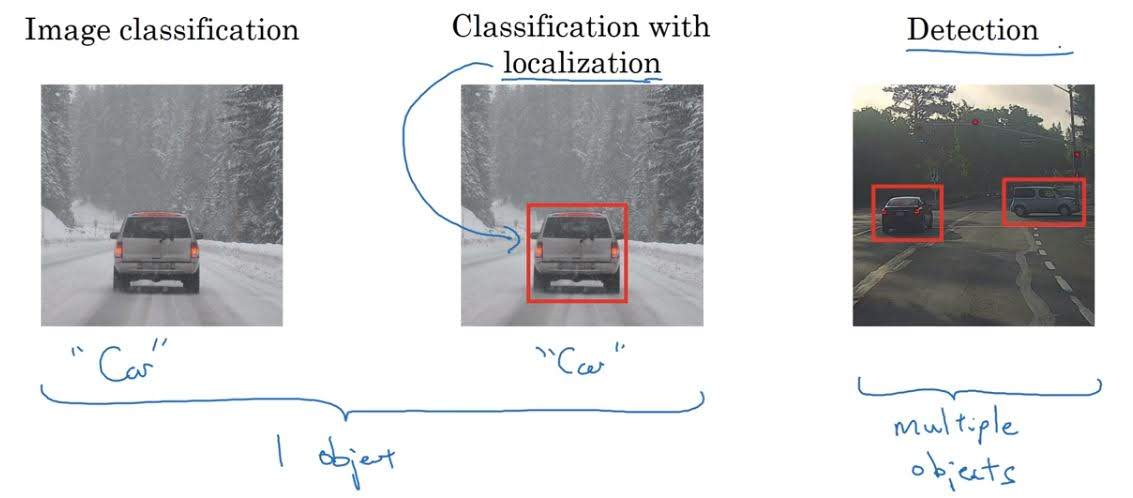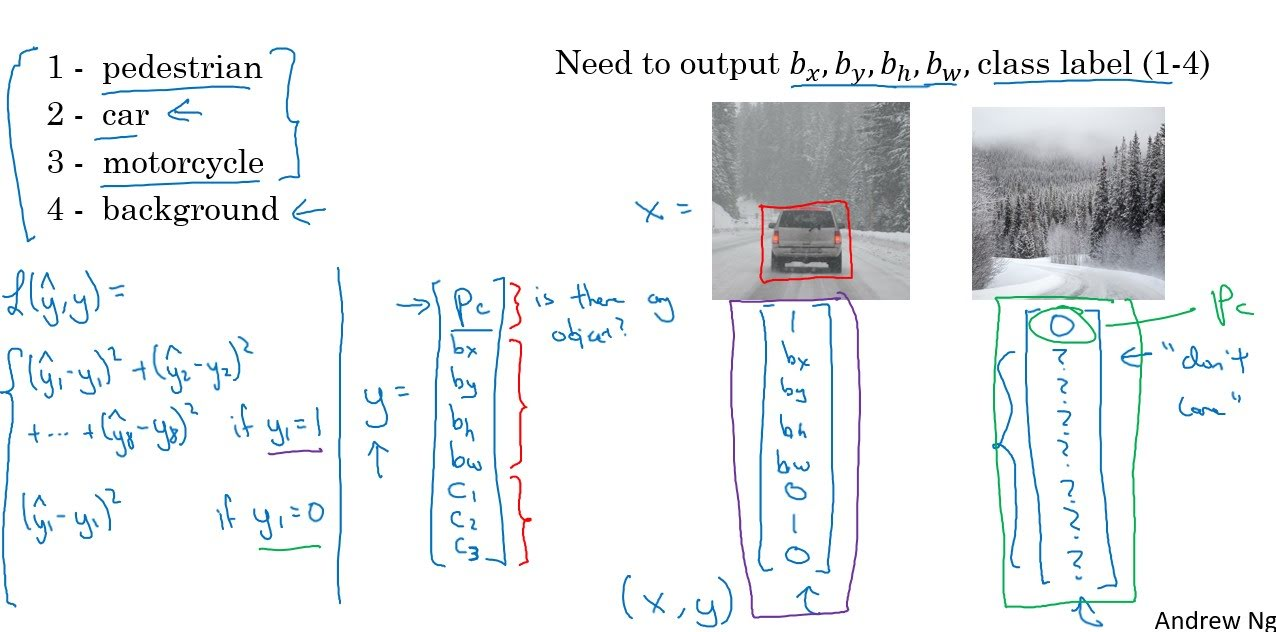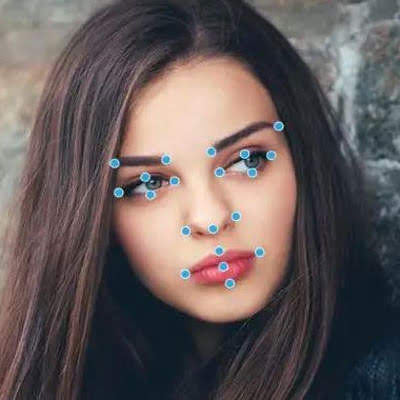Categories:

Updated:

# Differences Between Localization and DetectionObject classification with localization is a task where with an input image, you need to output one object class and location of that object.

On the other hand, in object detection you need to output multiple objects with different classes and location of that objects in an image.

# Output of Localization

With an input image, we should make the model that outputs three types of information;

1. Is there an object in the image?
2. If there is, where is the center of the object and what is the width, height of the object`?
3. If there is, in which class does the object belong?

Let’s say that we want to classify and localize three objects; pedestrian, car, and motorcycle. Then our output of one image should be a vector of 8 elements.

• First element will be 1 if there is an object in the image and 0 if there isn’t. ($p_c$)
• Second element represents the x-axis of the center of the object. ($b_x$)
• Third element represents the y-axis of the center of the object. ($b_y$)
• Fourth element represents the height of the object. ($b_h$)
• Fifth element represents the width of the object. ($b_w$)
• Sixth element will be 1 if the object is a pedestrian and 0 if it isn’t. ($c_1$)
• Seventh element will be 1 if the object is a car and 0 if it isn’t. ($c_2$)
• Eighth element will be 1 if the object is a motorcycle and 0 if it isn’t. ($c_3$)# Loss of Localization

Let’s say $K$ is the number of classes. (In the above example, $K=3$.)

$L(\hat{y},y)$

$=\sum_{k=1}^{K+5} (\hat{y_k}-y_k)^2$ if $y_1=1$.

$=(\hat{y_1}-y_1)^2$ if $y_1=0$.

Here we’ve calculated with mean squared loss but in practice, we can use binary cross entropy with $p_c$, mean squared error with $b$s, multiclass cross entropy with $c$s as loss functions.

# Landmark Detection

Landmark detection is basically the same as object localization. The only difference is that it outputs points, rather than area.

Let’s say you want to find out important points in human face (corners of the mouth and tip of the chin). What you have to do is train a CNN with output that is composed of x and y coordinates of these important points.

The output of one image will be a vector composed of 7 elements;

1. 1 if it is a face and 0 if it isn’t
2. x-coordinate of the left corner of the mouth
3. y-coordinate of the left corner of the mouth
4. x-coordinate of the right corner of the mouth
5. y-coordinate of the right corner of the mouth
6. x-coordinate of the tip of the chin
7. y-coordinate of the tip of the chin

Below is the example of landmark detection in face.Categories: